# Python机器学习笔记 使用scikit-learn工具进行PCA降维Python机器学习笔记 使用scikit-learn工具进行PCA降维### 一，PCA 的目的

PCA算法是一种在尽可能减少信息损失的前提下，找到某种方式降低数据的维度的方法。PCA通常用于高维数据集的探索与可视化，还可以用于数据压缩，数据预处理。

### 二，PCA算法思路

1，去掉数据的类别特征（label），将去掉后的 d 维数据作为样本

2，计算 d 维的均值向量（即所有数据的每一维向量的均值）

3，计算所有数据的散布矩阵（或者协方差矩阵）

4，计算特征值（e1 , e2 , e3 , …. ed）以及相应的特征向量（lambda1，lambda2，…lambda d）

5，按照特征值的大小对特征向量降序排序，选择前 k 个最大的特征向量，组成 d*k 维的矩阵W（其中每一列代表一个特征向量）

6，运行 d*K 的特征向量矩阵W将样本数据变换成新的子空间。

• 注意1：虽然PCA有降维的效果，也许对避免过拟合有作用，但是最好不要用PCA去作用于过拟合。
• 注意2：在训练集中找出PCA的主成分，（可以看做为映射mapping），然后应用到测试集和交叉验证集中，而不是对所有数据集使用PCA然后再划分训练集，测试集和交叉验证集。

### 三，PCA算法流程

1） 对有所的样本进行中心化：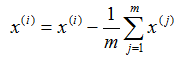2） 计算样本的协方差矩阵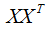3） 对矩阵进行特征值分解

4） 取出最大的 n 个特征值对应的特征向量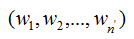，将所有的特征向量标准化后，组成特征向量矩阵W。

5） 对样本集中的每一个样本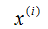，转化为新的样本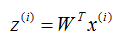6） 得到输出的样本集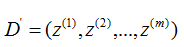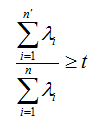### 四，PCA算法优缺点总结

PCA算法作为一个非监督学习的降维方法，它只需要特征值分解，就可以对数据进行压缩，去噪。因此在实际场景应用很广泛。为了克服PCA的一些缺点，出现了很多PCA的变种，比如未解决非线性降维的KPCA，还有解决内存限制的增量PCA方法Incremental PCA ，以及解决稀疏数据降维的PCA方法Sparse PCA 等等。

#### 4.1  PCA算法优点

1，仅仅需要以方差衡量信息量，不受数据集以外的因素影响

2，各主成分之间正交，可消除原始数据成分间的相互影响的因素

3，计算方法简单，主要运算时特征值分解，易于实现

#### 4.2  PCA算法缺点

1，主成分各个特征维度的含义具有一定的模糊性，不如原始样本特征的解释性强

2，方差小的非主成分也可能含有对样本差异的重要信息，因降维丢弃可能对后续数据处理有影响。

## scikit-learn PCA类介绍

 1 2 3 4 5 6 7 8 9 10 11 12 13 14 15 16 17 18 19 20 21 22 23 24 25 26 27 28 29 30 31 32 33 34 35 36 37 38 39 40 """ The :mod:sklearn.decomposition module includes matrix decomposition algorithms, including among others PCA, NMF or ICA. Most of the algorithms of this module can be regarded as dimensionality reduction techniques. """   from .nmf import NMF, non_negative_factorization from .pca import PCA, RandomizedPCA from .incremental_pca import IncrementalPCA from .kernel_pca import KernelPCA from .sparse_pca import SparsePCA, MiniBatchSparsePCA from .truncated_svd import TruncatedSVD from .fastica_ import FastICA, fastica from .dict_learning import (dict_learning, dict_learning_online, sparse_encode,                             DictionaryLearning, MiniBatchDictionaryLearning,                             SparseCoder) from .factor_analysis import FactorAnalysis from ..utils.extmath import randomized_svd from .online_lda import LatentDirichletAllocation   __all__ = ['DictionaryLearning',            'FastICA',            'IncrementalPCA',            'KernelPCA',            'MiniBatchDictionaryLearning',            'MiniBatchSparsePCA',            'NMF',            'PCA',            'RandomizedPCA',            'SparseCoder',            'SparsePCA',            'dict_learning',            'dict_learning_online',            'fastica',            'non_negative_factorization',            'randomized_svd',            'sparse_encode',            'FactorAnalysis',            'TruncatedSVD',            'LatentDirichletAllocation']

### 一，sklearn.decomposition.PCA 参数介绍

1）n_components：这个参数可以帮我们指定希望PCA降维后的特征维度数目。最常用的做法是直接指定降维到的维度数目，此时n_components是一个大于等于1的整数。当然，我们也可以指定主成分的方差和所占的最小比例阈值，让PCA类自己去根据样本特征方差来决定降维到的维度数，此时n_components是一个（0，1]之间的数。当然，我们还可以将参数设置为”mle”, 此时PCA类会用MLE算法根据特征的方差分布情况自己去选择一定数量的主成分特征来降维。我们也可以用默认值，即不输入n_components，此时n_components=min(样本数，特征数)。

2）whiten ：判断是否进行白化。所谓白化，就是对降维后的数据的每个特征进行归一化，让方差都为1.对于PCA降维本身来说，一般不需要白化。如果你PCA降维后有后续的数据处理动作，可以考虑白化。默认值是False，即不进行白化。

3）svd_solver：即指定奇异值分解SVD的方法，由于特征分解是奇异值分解SVD的一个特例，一般的PCA库都是基于SVD实现的。有4个可以选择的值：{‘auto’, ‘full’, ‘arpack’, ‘randomized’}。randomized一般适用于数据量大，数据维度多同时主成分数目比例又较低的PCA降维，它使用了一些加快SVD的随机算法。 full则是传统意义上的SVD，使用了scipy库对应的实现。arpack和randomized的适用场景类似，区别是randomized使用的是scikit-learn自己的SVD实现，而arpack直接使用了scipy库的sparse SVD实现。默认是auto，即PCA类会自己去在前面讲到的三种算法里面去权衡，选择一个合适的SVD算法来降维。一般来说，使用默认值就够了。

### 二，PCA对象的属性

components_ ：返回具有最大方差的成分。

explained_variance_ratio_ ：返回所保留的 n 个成分各自的方差百分比

n_components_：返回所保留的成分个数 n

mean_ ：

noise_variance_ ：

### 三，PCA对象的方法

1） fit(X, y=None)  fit() 可以说是scikit-learn中通用的方法，每个需要训练的算法都会有fit()方法，他其实就是算法中的“训练”这一步骤。因为PCA是无监督学习算法，此处y自然等于None。fit(X)，表示用数据 X 来训练PCA模型。函数返回值：调用fit方法的对象本身。比如pca.fit(X)，表示用X对pca这个对象进行训练。

2）fit_transform(X)  用X来训练PCA模型，同时返回降维后的数据， NewX = pca.fit_transform(X)。NewX就是降维后的数据。

3）inverse_transform()  将降维后的数据转换成原始数据，X = pca.inverse_transform(NewX)

4）tranform(X) 将数据X转换成降维后的数据。当模型训练好后，对于新输入的数据，都可以用transform 方法来降维。

### 四，PCA实例1

#### 1，首先生成随机数据并可视化

 1 2 3 4 5 6 7 8 9 10 11 12 13 14 15 16 import numpy as np import matplotlib.pyplot as plt from mpl_toolkits.mplot3d import Axes3D # make_nlobs方法常被用来生成聚类算法的测试数据 # make_blobs会根据用户指定的特征数量，中心点数量，范围等来生成几类数据 from sklearn.datasets.samples_generator import make_blobs # X为样本特征，Y为样本簇类型 共10000个样本，每个样本3个特征，共4个簇 # n_samples表示产生多少个数据  n_features表示数据是几维， # centers表示中心点 cluster_std表示分布的标准差 X, y = make_blobs(n_samples=10000, n_features=3, centers=[[3, 3, 3], [0, 0, 0], [1, 1, 1],                 [2, 2, 2]], cluster_std=[0.2, 0.1, 0.2, 0.2], random_state=9) fig = plt.figure() ax = Axes3D(fig, rect=[0, 0, 1, 1], elev=30, azim=20) plt.scatter(X[:, 0], X[:, 1], X[:, 2], marker='o') plt.show()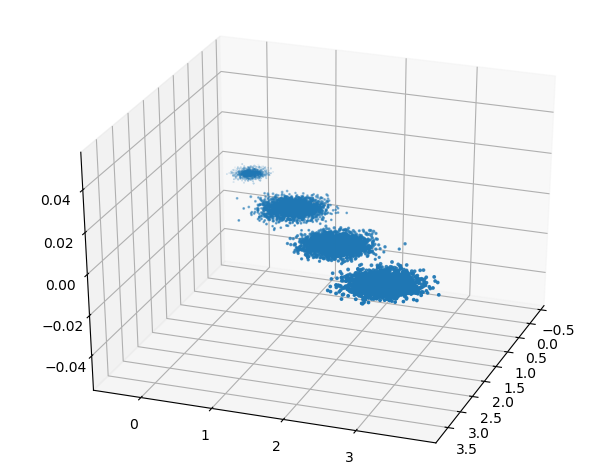1 2 3 4 5 6 from sklearn.decomposition import PCA pca = PCA(n_components=3) pca.fit(X) print(pca.explained_variance_ratio_) print(pca.explained_variance_)

 1 2 3 [0.98318212 0.00850037 0.00831751] [3.78521638 0.03272613 0.03202212]

 1 2 3 4 5 6 from sklearn.decomposition import PCA pca = PCA(n_components=2) pca.fit(X) print(pca.explained_variance_ratio_) print(pca.explained_variance_)

 1 2 3 [0.98318212 0.00850037] [3.78521638 0.03272613]

 1 2 3 X_new = pca.transform(X) plt.scatter(X_new[:, 0], X_new[:, 1],marker='o') plt.show()

（报错待学习）

 1 2 3 4 5 pca = PCA(n_components=0.95) pca.fit(X) print(pca.explained_variance_ratio_) print(pca.explained_variance_) print(pca.n_components_)

 1 2 3 [0.98318212] [3.78521638] 1

 1 2 3 4 5 pca = PCA(n_components = 0.99) pca.fit(X) print(pca.explained_variance_ratio_) print(pca.explained_variance_) print(pca.n_components_)

 1 2 3 [0.98318212 0.00850037] [3.78521638 0.03272613] 2

 1 2 3 4 5 pca = PCA(n_components = 'mle') pca.fit(X) print(pca.explained_variance_ratio_) print(pca.explained_variance_) print(pca.n_components_)

 1 2 3 [0.98318212] [3.78521638] 1

### 五，IRIS数据集实践IPCA（Incremental  PCA）

IRIS数据集是常见的分类试验数据集，也成为鸢尾花数据集，是一类多重变量分析的数据集。数据集包含150个数据集，分为三类，没类50个数据，每个数据包含4个特征。可以通过花萼长度，花萼宽度，花瓣长度，花瓣宽度（sepal length，sepal width ，petal length ，petal width ）4个特征预测鸢尾花卉属于（Setosa，Versicolour，Virginica）三个种类中的哪一类。

#### 1，选取三个特征查看数据分布情况

 1 2 3 4 5 6 7 8 9 10 11 12 13 14 15 16 17 18 19 #_*_coding:utf-8_*_ import matplotlib.pyplot as plt from mpl_toolkits.mplot3d import Axes3D from sklearn import datasets data = datasets.load_iris() X =data['data'] y =data['target'] ax = Axes3D(plt.figure()) for c, i, target_name in zip('rgb', [0, 1, 2], data.target_names):     ax.scatter(X[y==i, 0], X[y==i, 2], c=c, label=target_name) ax.set_xlabel(data.feature_names) ax.set_xlabel(data.feature_names) ax.set_xlabel(data.feature_names) ax.set_title('Iris') plt.legend() plt.show()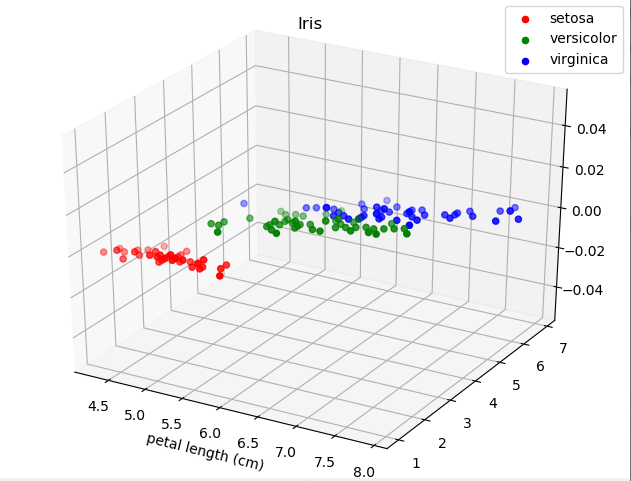#### 2，选取两个特征查看数据分布情况

 1 2 3 4 5 6 7 8 9 10 11 12 13 14 15 16 17 18 #_*_coding:utf-8_*_ import matplotlib.pyplot as plt from mpl_toolkits.mplot3d import Axes3D from sklearn import datasets data = datasets.load_iris() X =data['data'] y =data['target'] ax = Axes3D(plt.figure()) for c, i, target_name in zip('rgb', [0, 1, 2], data.target_names):     ax.scatter(X[y==i, 0], X[y==i, 1], c=c, label=target_name) ax.set_xlabel(data.feature_names) ax.set_xlabel(data.feature_names) ax.set_title('Iris') plt.legend() plt.show()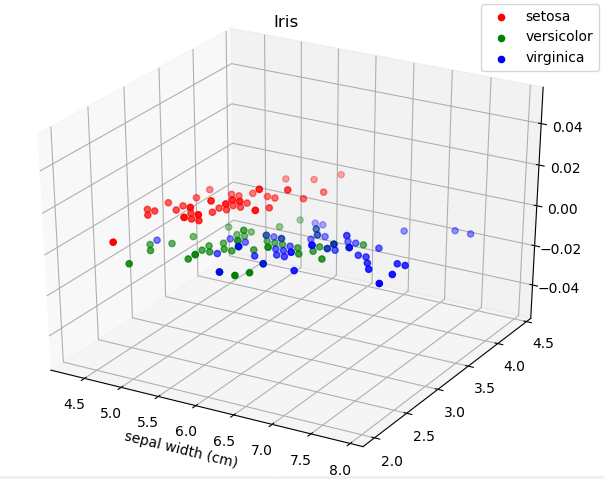#### 3，利用PCA降维，降到二维

 1 2 3 4 5 6 7 8 9 10 11 12 13 14 15 16 17 18 19 20 #_*_coding:utf-8_*_ import matplotlib.pyplot as plt from mpl_toolkits.mplot3d import Axes3D from sklearn import datasets from sklearn.decomposition import PCA data = datasets.load_iris() X =data['data'] y =data['target'] pca = PCA(n_components=2) X_p = pca.fit(X).transform(X) ax = plt.figure() for c, i, target_name in zip('rgb', [0, 1, 2], data.target_names):     plt.scatter(X_p[y==i, 0], X_p[y==i, 1], c=c, label=target_name) plt.xlabel('Dimension1') plt.ylabel('Dimension2') plt.title('Iris') plt.legend() plt.show()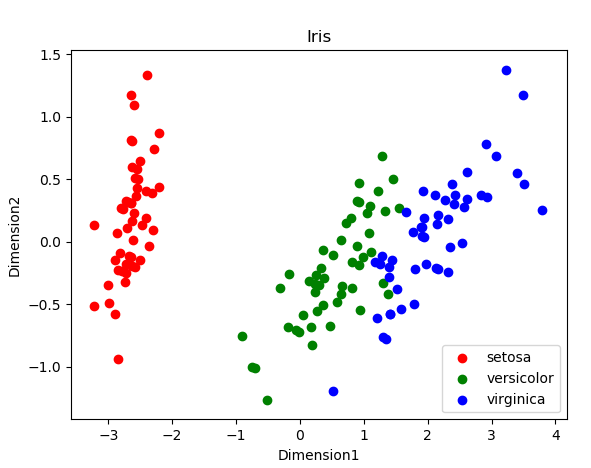#### 4，利用pandas库查看数据分析

pandas数据可以理解为一种结构化的表格数据

 1 2 3 4 5 6 7 8 9 10 11 12 13 14 15 16 17 18 19 #_*_coding:utf-8_*_ import matplotlib.pyplot as plt from mpl_toolkits.mplot3d import Axes3D from sklearn import datasets from sklearn.decomposition import PCA import pandas as pd import seaborn data = datasets.load_iris() X =data['data'] y =data['target'] a = pd.DataFrame(X, columns=data.feature_names) # 随机打印一下看看数据 print(a.sample(5)) # 箱图，看数据范围 seaborn.boxplot(data= a) plt.plot(a) plt.legend(data.feature_names) plt.show()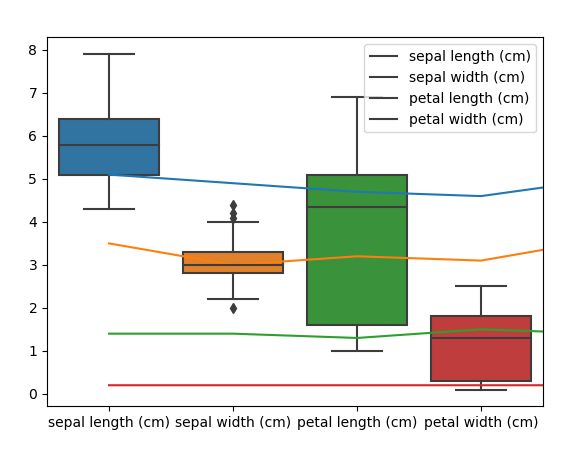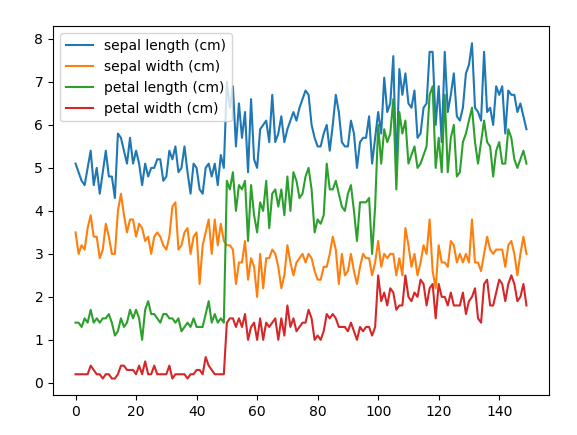#### 5，Incremental PCA

IPCA 是将数据分成多个batch，然后对每个batch依次递增调用partial_fit函数，这样一步步的得到最终的样本最优降维。

 1 2 3 4 5 6 7 8 9 10 11 12 13 14 15 16 17 18 19 20 21 22 23 24 25 26 27 28 29 30 31 32 33 34 import numpy as np import matplotlib.pyplot as plt from sklearn.datasets import load_iris from sklearn.decomposition import PCA , IncrementalPCA iris = load_iris() X = iris.data y = iris.target n_components = 2 ipca = IncrementalPCA(n_components=n_components, batch_size=10) X_ipca = ipca.fit_transform(X) pca = PCA(n_components=n_components) X_pca = pca.fit_transform(X) colors = ['navy', 'turquoise', 'darkorange'] for X_trainsformed, title in [(X_ipca, "Incremental PCA"), (X_pca, "PCA")]:     plt.figure(figsize=(8, 8))     for color, i ,target_name in zip(colors, [0,1,2], iris.target_names):         plt.scatter(X_trainsformed[y == i, 0], X_trainsformed[y ==i, 1],                     color=color, lw=2, label=target_name)     if "Incremental" in title:         err = np.abs(np.abs(X_pca) - np.abs(X_ipca)).mean()         plt.title(title + 'of iris dataset\nMean absolute unsigned error%.6f'%err)     else:         plt.title(title + 'of iris dataset')     plt.legend(loc='best', shadow=False, scatterpoints=1)     plt.axis([-4, 4, -1.5, 1.5]) plt.show()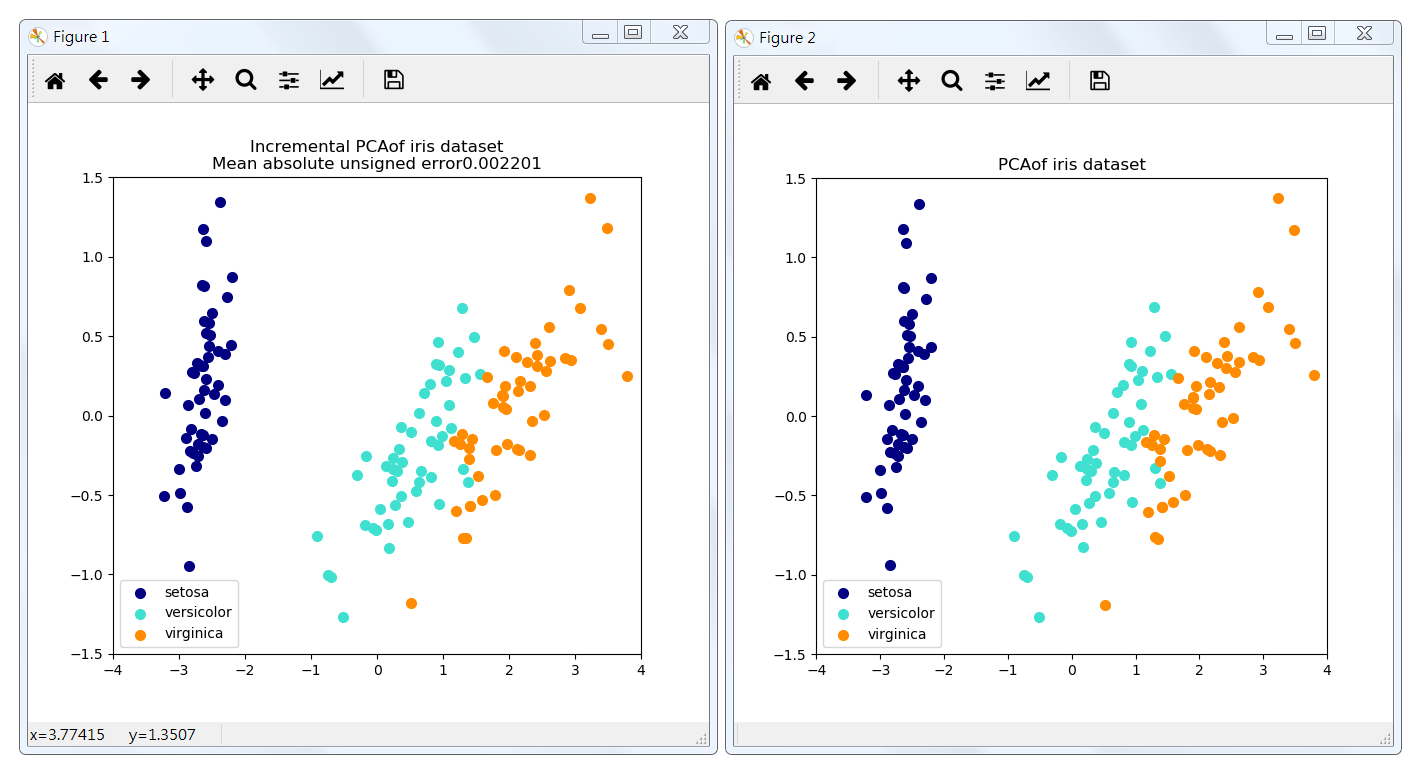#### 6，Kernel PCA

 1 2 3 4 5 6 7 8 9 10 11 12 13 14 15 16 17 18 19 20 21 22 23 24 25 26 27 28 29 30 31 32 33 34 35 36 37 38 39 40 41 42 43 44 45 46 47 48 49 50 51 52 53 54 55 56 57 58 59 60 61 62 63 64 65 66 import numpy as np import matplotlib.pyplot as plt from sklearn.decomposition import PCA, KernelPCA from sklearn.datasets import make_circles np.random.seed(0) X, y = make_circles(n_samples=400, factor=0.3, noise=0.05) kpca = KernelPCA(kernel='rbf', fit_inverse_transform=True, gamma=10) X_kpca = kpca.fit_transform(X) X_back = kpca.inverse_transform(X_kpca) pca = PCA() X_pca = pca.fit_transform(X) # Plot results plt.figure() plt.subplot(2, 2, 1, aspect='equal') plt.title("Original space") reds = y == 0 blues = y == 1 plt.scatter(X[reds, 0], X[reds, 1], c="red",             s=20, edgecolor='k') plt.scatter(X[blues, 0], X[blues, 1], c="blue",             s=20, edgecolor='k') plt.xlabel("$x_1$") plt.ylabel("$x_2$") X1, X2 = np.meshgrid(np.linspace(-1.5, 1.5, 50), np.linspace(-1.5, 1.5, 50)) X_grid = np.array([np.ravel(X1), np.ravel(X2)]).T # projection on the first principal component (in the phi space) Z_grid = kpca.transform(X_grid)[:, 0].reshape(X1.shape) plt.contour(X1, X2, Z_grid, colors='grey', linewidths=1, origin='lower') plt.subplot(2, 2, 2, aspect='equal') plt.scatter(X_pca[reds, 0], X_pca[reds, 1], c="red",             s=20, edgecolor='k') plt.scatter(X_pca[blues, 0], X_pca[blues, 1], c="blue",             s=20, edgecolor='k') plt.title("Projection by PCA") plt.xlabel("1st principal component") plt.ylabel("2nd component") plt.subplot(2, 2, 3, aspect='equal') plt.scatter(X_kpca[reds, 0], X_kpca[reds, 1], c="red",             s=20, edgecolor='k') plt.scatter(X_kpca[blues, 0], X_kpca[blues, 1], c="blue",             s=20, edgecolor='k') plt.title("Projection by KPCA") plt.xlabel(r"1st principal component in space induced by $\phi$") plt.ylabel("2nd component") plt.subplot(2, 2, 4, aspect='equal') plt.scatter(X_back[reds, 0], X_back[reds, 1], c="red",             s=20, edgecolor='k') plt.scatter(X_back[blues, 0], X_back[blues, 1], c="blue",             s=20, edgecolor='k') plt.title("Original space after inverse transform") plt.xlabel("$x_1$") plt.ylabel("$x_2$") plt.subplots_adjust(0.02, 0.10, 0.98, 0.94, 0.04, 0.35) plt.show()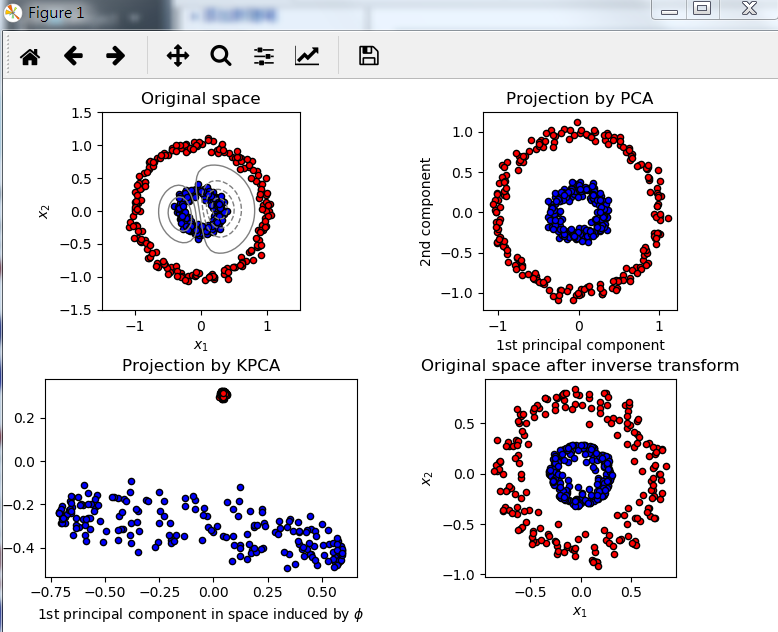### 知识扩展：python 中的 __doc__是什么意思？

（对于代码功能的注释，通过__doc__ 可以输出）

1， 一个文件任何一条可执行的代码之前   # 模块的__doc__

2， 一个类，在类定于语句后，任何可执行代码前   # 类的 __doc__

3，一个函数，在函数定于语句后，任何可执行代码前   # 函数的__doc__

 1 2 3 4 5 6 7 8 9 10 11 12 13 14 15 # use  __doc__ 属性 class MyClass:     'string.'     def printSay(self):         'print say welcome to you.'         print('say welcome to you.') print(MyClass.__doc__) print(MyClass.printSay.__doc__) # 输出结果 string. print say welcome to you.

https://www.cnblogs.com/pinard/p/6239403.html• 回顶